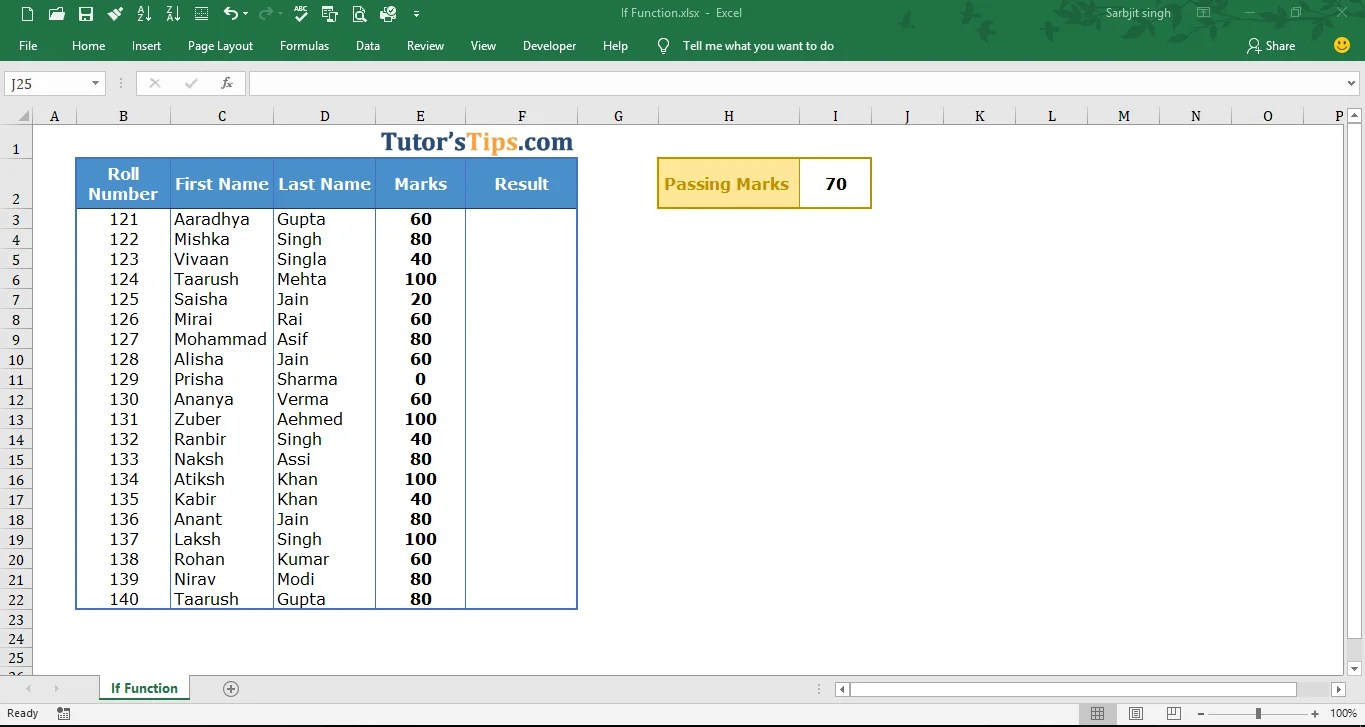# IF Function in Excel – Explanation with example## IF Function in Excel: –

The “IF” Function in Excel is a logical test, which represents the result in two way if the logical condition true and false. There is always two way of getting the value, one is when we got our condition/logic true and another we will get when the condition/logic false. We can use this formula where we want to apply the condition of less than, greater than and equal to. It means if the value of the selected cell is less than from specific amount then answer the specific true value(it may be in numeric or in words) rather then answer the false value.

### The feature of “IF” function: –

• With the help of IF Function, we can retrieve the data if the condition is cleared by the data.
• VLOOKUP and HLOOKUP shows only matched value but “IF” function shows the value in both ways.
• It helps in applying a particular condition on the data.

### The logical operator: –

The “IF” function we can use only follow logical test on the selected data.

 Logical Test Meaning = Equal to <> Not Equal to > Greater than >= Greater than and Equal to < Less than <= Less than and equal to

### Explanation of Function:-

Now, We will explain the Arguments of the Function.

=IF(logical_test, [value_if_true], [value_if_false])

logical_test:- The Logical test is the value or condition which will provide us with the result of the selected cell as true or false. It is a specific condition/test as explained in the above table.

value_if_true: – The value if true is that value which you want to show if the condition or logical test is true.

value_if_false: – The value if false is that value which you want to show if the condition or logical test is false.

### Example of Function: –

In the following table, we want to retrieve the result of all students in Pass and Fail Form. The minimum passing marks are 70.Solution:-

We will Apply the if function and get the result. This is shown in the following steps: –

Step No. 1:-

We will write the “=if( ” in the column of the result.

Step No. 2

Now select the cell on which you want to apply the logical test. and then write the logical test

Or if can by selecting the cell where the value of logic is written and then fix this cell by adding “\$” signs.

Step No. 3: –

Now, write the answer which you want if the condition show when the condition is true. Write the answer in t invited comma.

Step No. 4: –

Now, write the answer which you want if the condition show when the condition is false. Write the answer in t invited comma.

Step No. 5: –

Now, Copy and paste the function in all remaining cell to get the result.